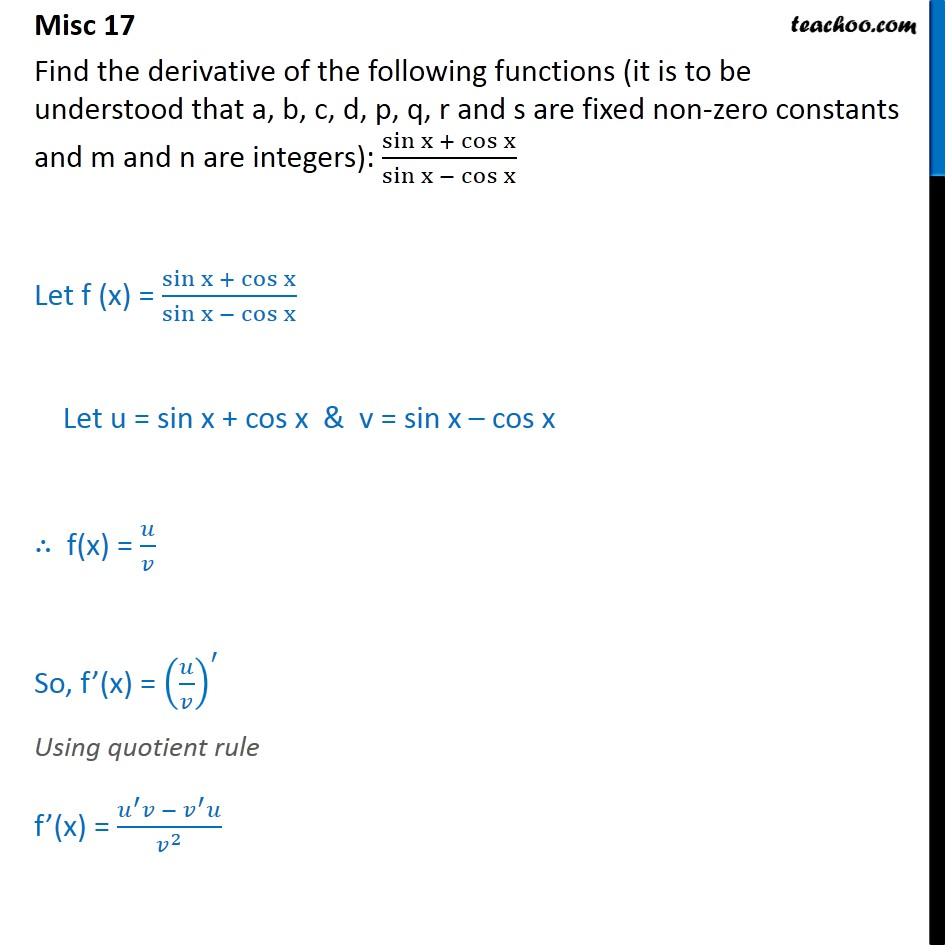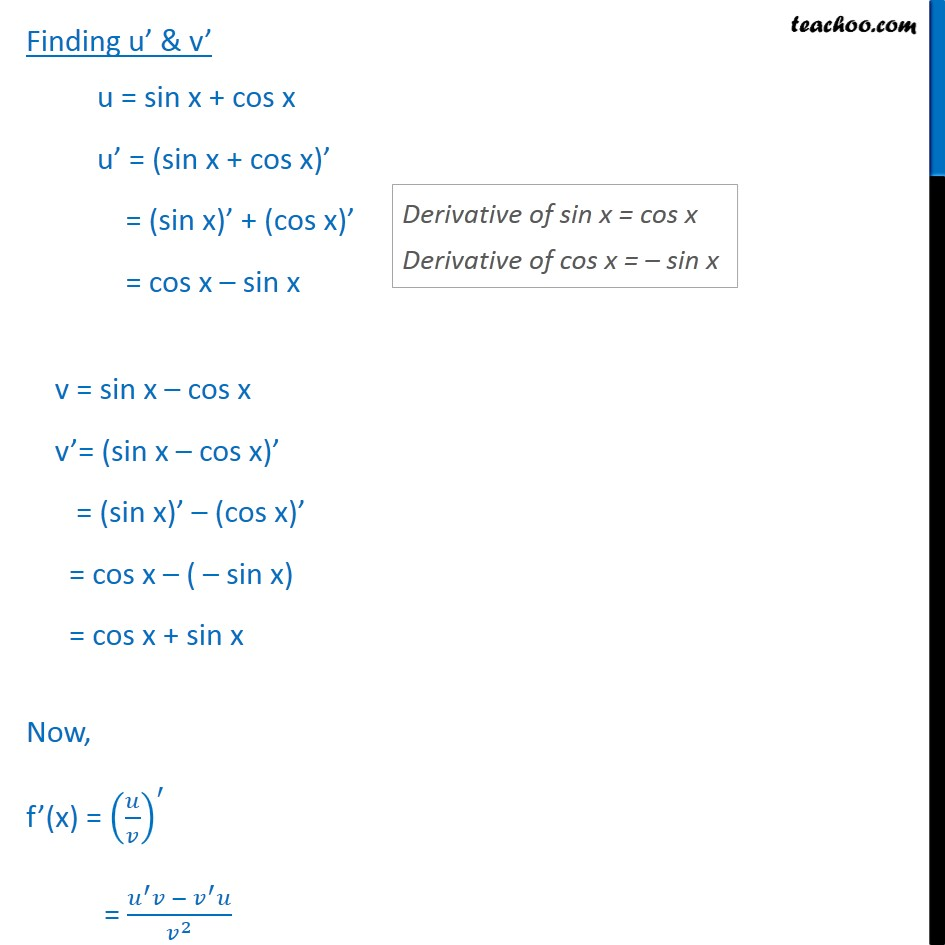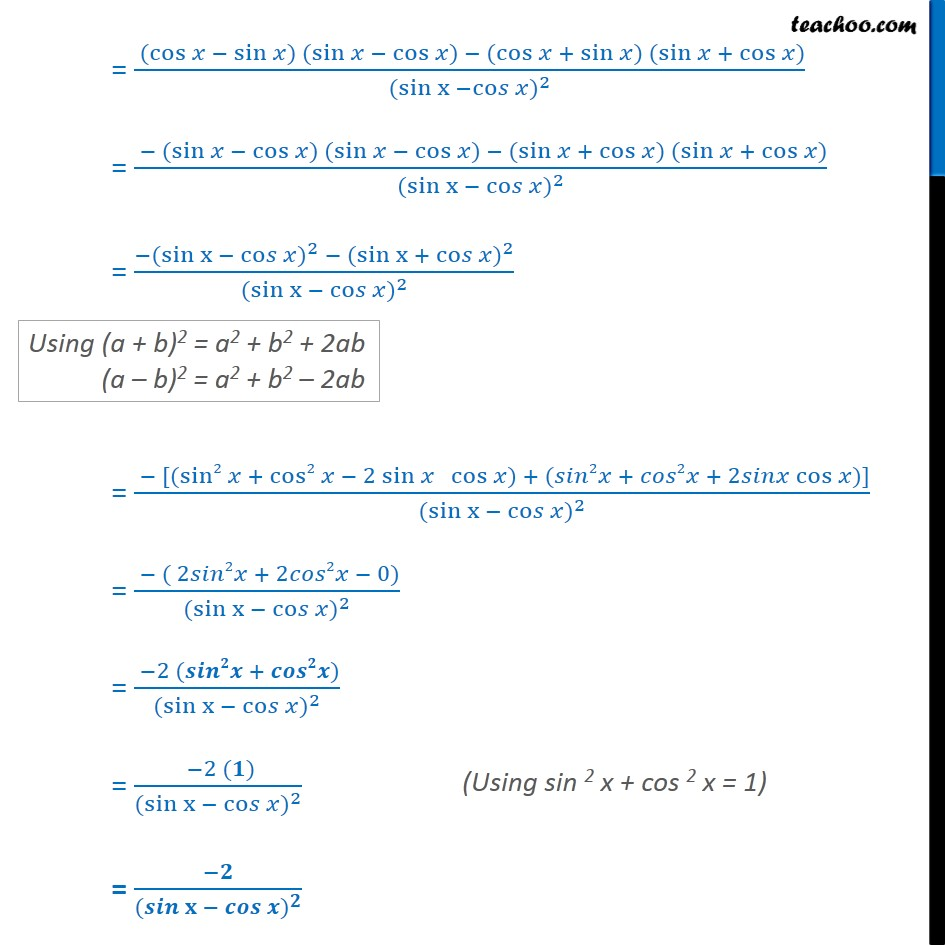1. Chapter 13 Class 11 Limits and Derivatives
2. Serial order wise
3. Miscellaneous

Transcript

Misc 17 Find the derivative of the following functions (it is to be understood that a, b, c, d, p, q, r and s are fixed non-zero constants and m and n are integers): sin﷮x + cos﷮x﷯﷯﷮ sin﷮x − cos﷮x﷯﷯﷯ Let f (x) = sin﷮x + cos﷮x﷯﷯﷮ sin﷮x − cos﷮x﷯﷯﷯ Let u = sin x + cos x & v = sin x – cos x ∴ f(x) = 𝑢﷮𝑣﷯ So, f’(x) = 𝑢﷮𝑣﷯﷯﷮′﷯ Using quotient rule f’(x) = 𝑢﷮′﷯𝑣 − 𝑣﷮′﷯𝑢﷮ 𝑣﷮2﷯﷯ Finding u’ & v’ u = sin x + cos x u’ = (sin x + cos x)’ = (sin x)’ + (cos x)’ = cos x – sin x v = sin x – cos x v’= (sin x – cos x)’ = (sin x)’ – (cos x)’ = cos x – ( – sin x) = cos x + sin x Now, f’(x) = 𝑢﷮𝑣﷯﷯﷮′﷯ = 𝑢﷮′﷯𝑣 − 𝑣﷮′﷯𝑢﷮ 𝑣﷮2﷯﷯ = ( cos﷮𝑥 − sin﷮𝑥) ( sin﷮𝑥 − cos﷮𝑥) − ( cos﷮𝑥 + sin﷮𝑥) ( sin﷮𝑥 + cos﷮𝑥)﷯﷯﷯﷯﷯﷯﷯﷯﷮ ( sin﷮x −co𝑠 𝑥﷯)﷮2﷯﷯ = − ( sin﷮𝑥 − cos﷮𝑥) ( sin﷮𝑥 − cos﷮𝑥) − ( sin﷮𝑥 + cos﷮𝑥) ( sin﷮𝑥 + cos﷮𝑥)﷯﷯﷯﷯﷯﷯﷯﷯﷮ ( sin﷮x − co𝑠 𝑥﷯)﷮2﷯﷯ = −( sin﷮x − co𝑠 𝑥﷯)﷮2﷯ − ( sin﷮x + co𝑠 𝑥﷯)﷮2﷯﷮ ( sin﷮x − co𝑠 𝑥﷯)﷮2﷯﷯ = − [( sin2﷮𝑥 + cos2﷮𝑥 − 2 sin﷮𝑥 cos﷮𝑥) + (𝑠𝑖𝑛2𝑥 + 𝑐𝑜𝑠2𝑥 + 2𝑠𝑖𝑛𝑥 cos﷮𝑥)]﷯﷯﷯﷯﷯﷮ ( sin﷮x − co𝑠 𝑥﷯)﷮2﷯﷯ = − ( 2𝑠𝑖𝑛2𝑥 + 2𝑐𝑜𝑠2𝑥 − 0)﷮ ( sin﷮x − co𝑠 𝑥﷯)﷮2﷯﷯ = −2 (𝒔𝒊𝒏𝟐𝒙 + 𝒄𝒐𝒔𝟐𝒙)﷮ ( sin﷮x − co𝑠 𝑥﷯)﷮2﷯﷯ = −2 (𝟏)﷮ ( sin﷮x − co𝑠 𝑥﷯)﷮2﷯﷯ = −𝟐 ﷮ ( 𝒔𝒊𝒏﷮𝐱 − 𝒄𝒐𝒔 𝒙﷯)﷮𝟐﷯﷯

Miscellaneous